SAT Math 1 and 2Home   Algebra   Geometry   Arithmetic   Trigonometry   Probability/Statistics   Calculus   Mechanics GED   SAT   ACT   College Mathematics   GRE   School Supplies   About Us

SAT Math 1 & 2

 Electronic Textbooks TDownloads - Preview, Get, Practice!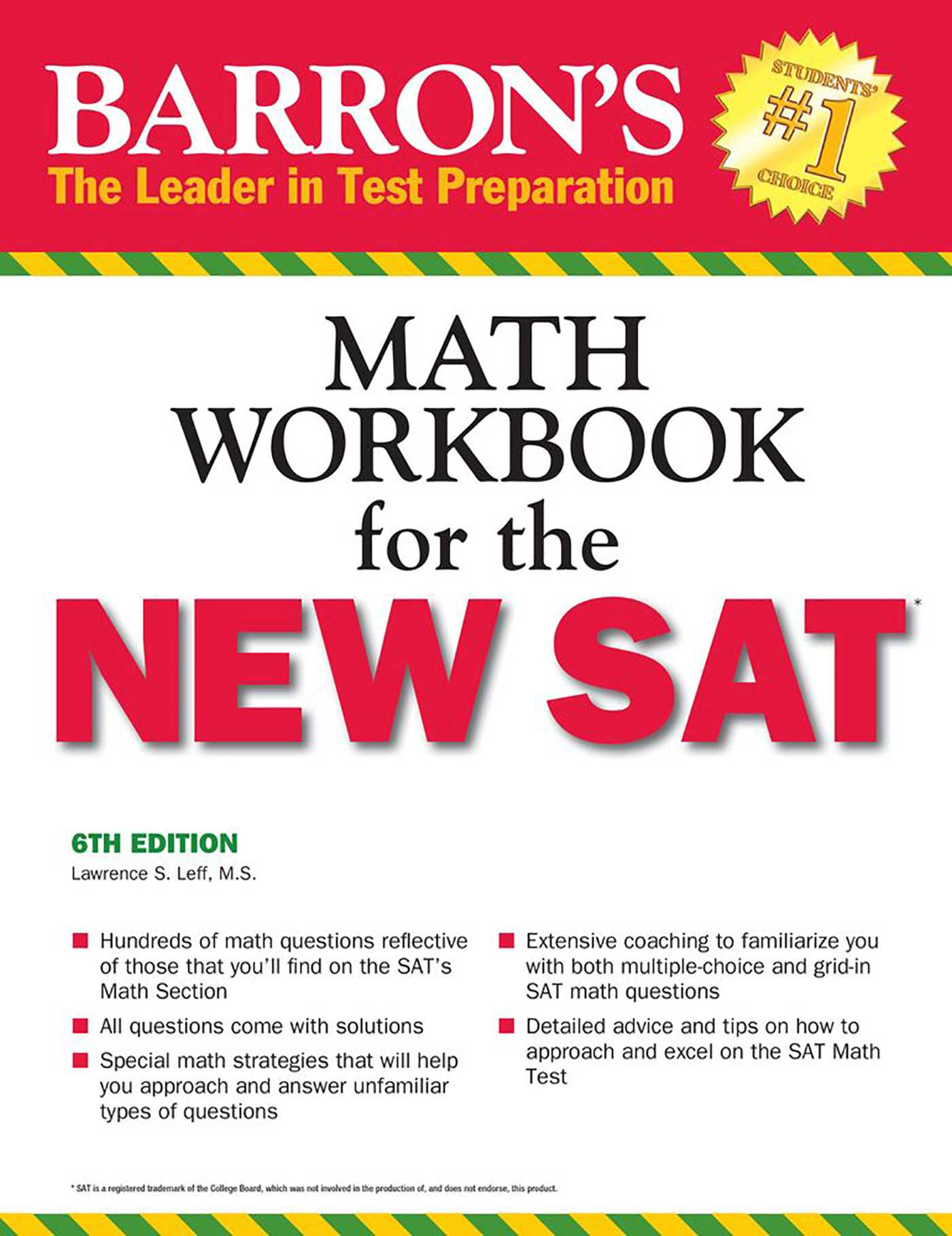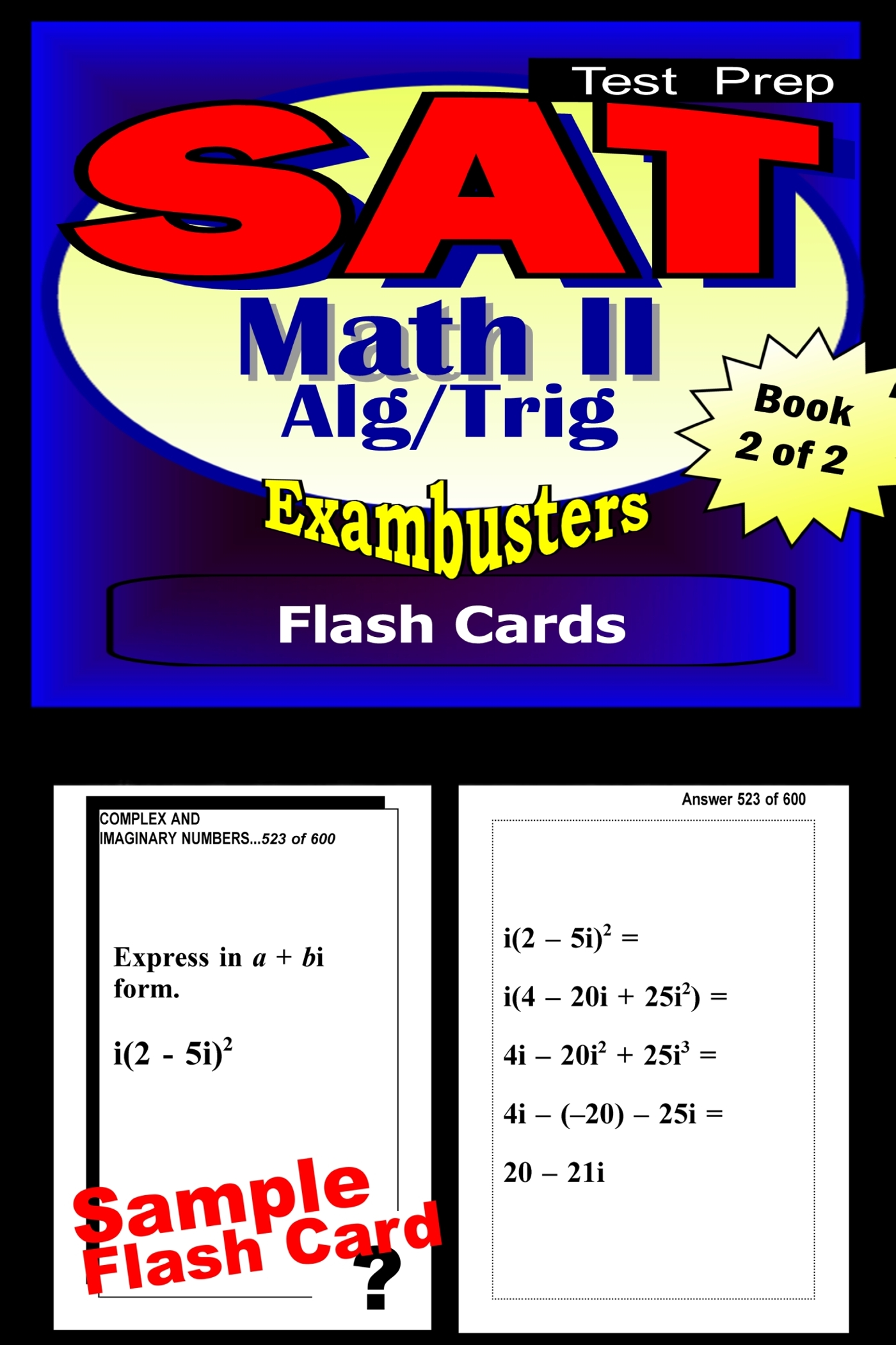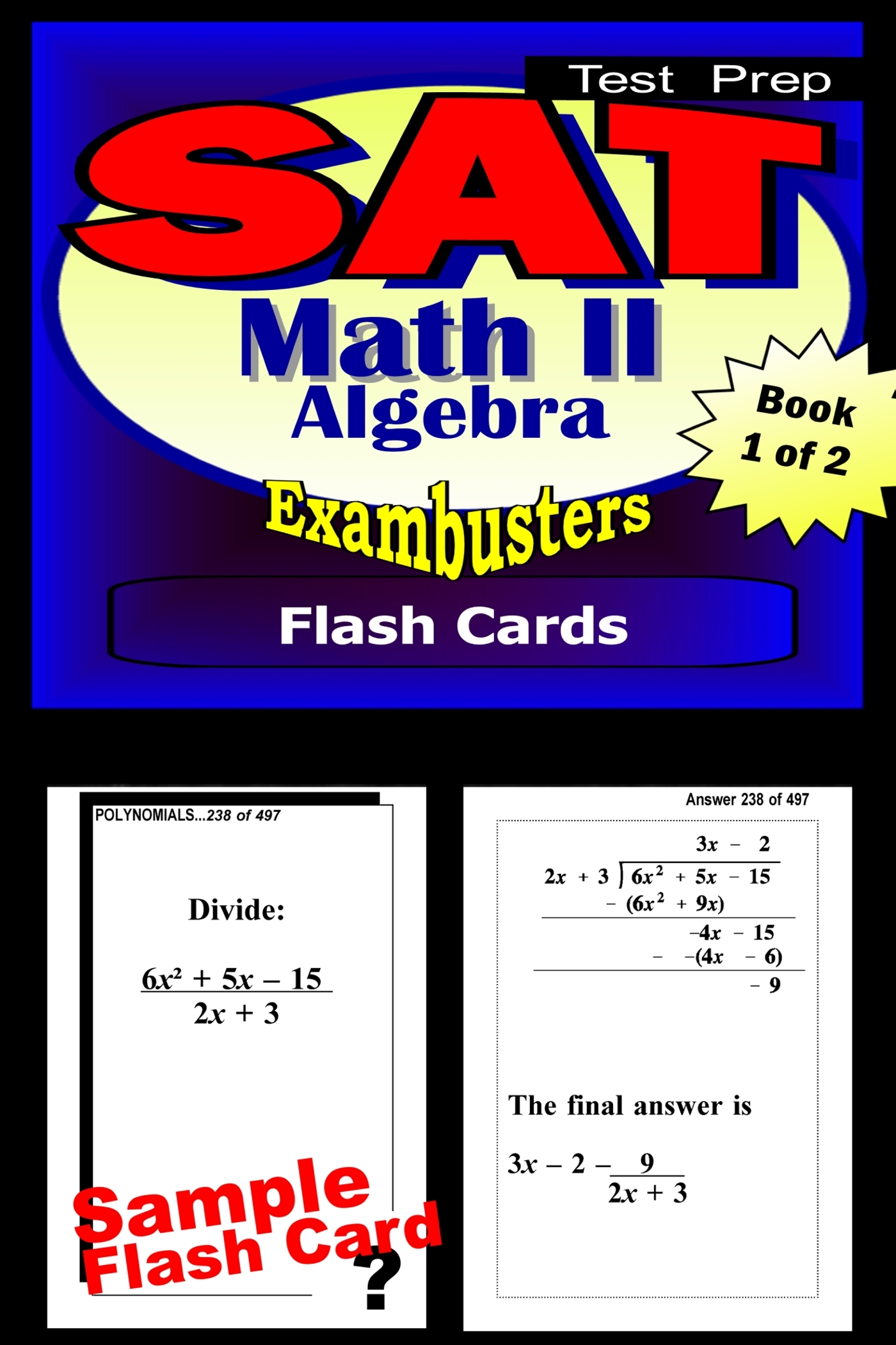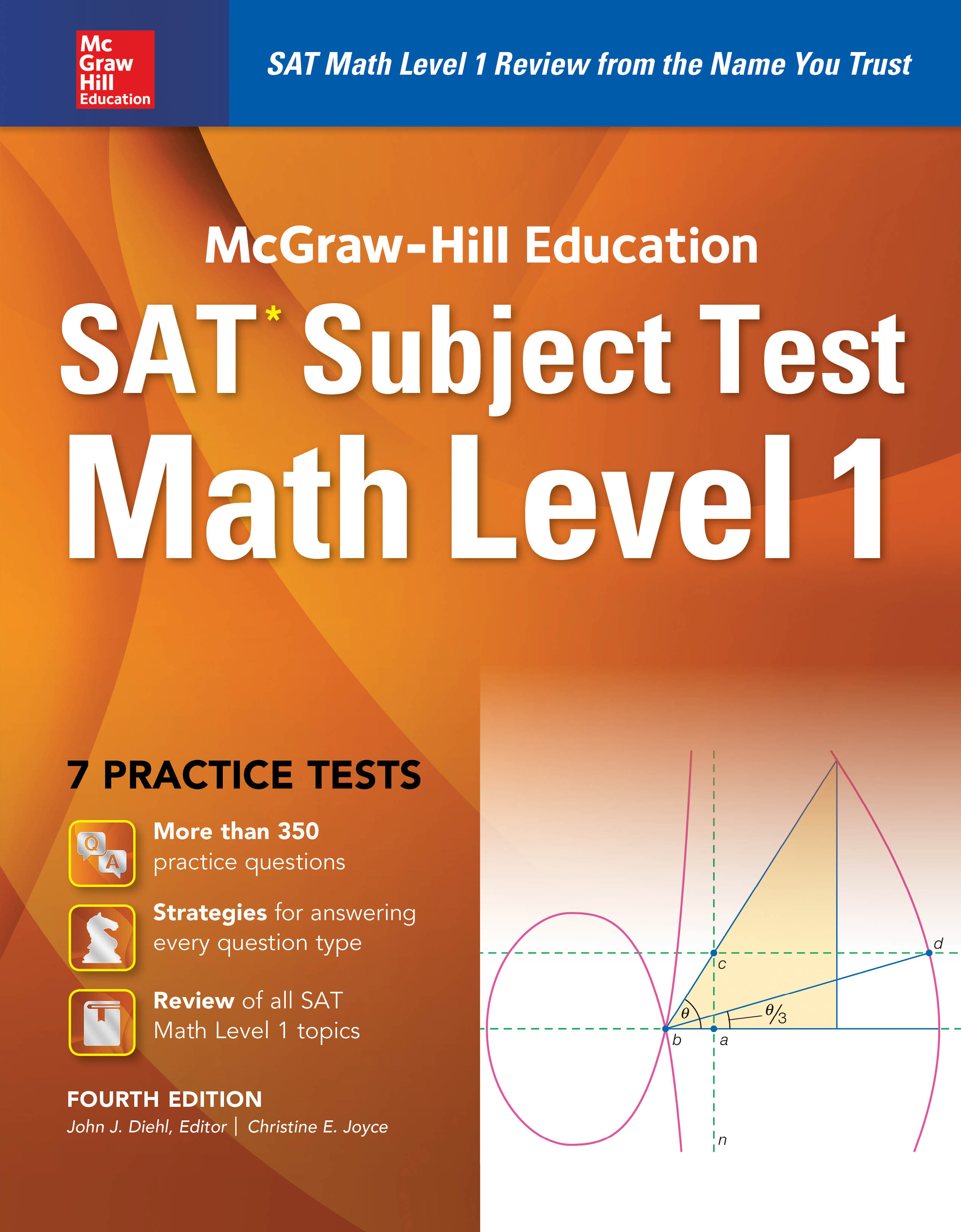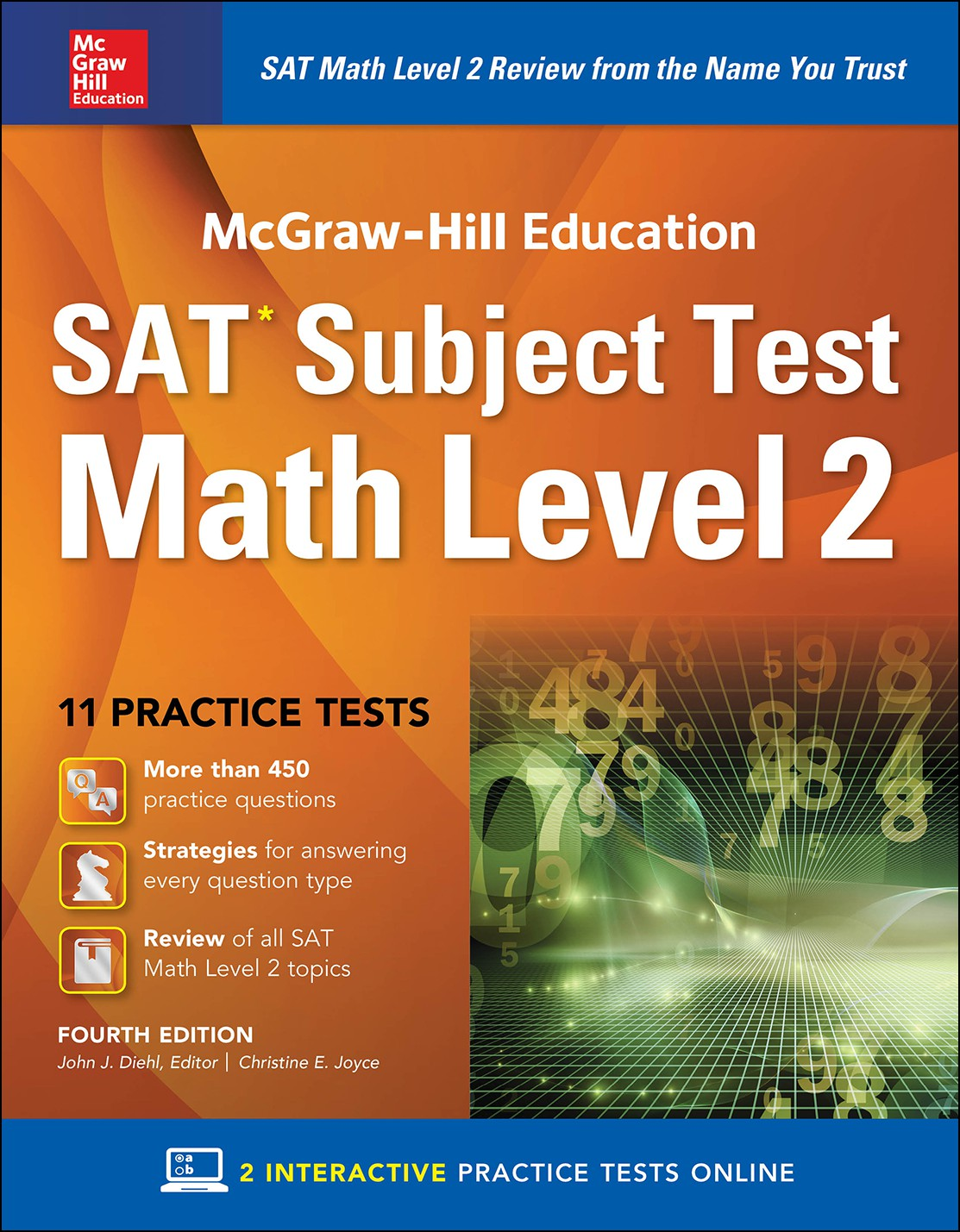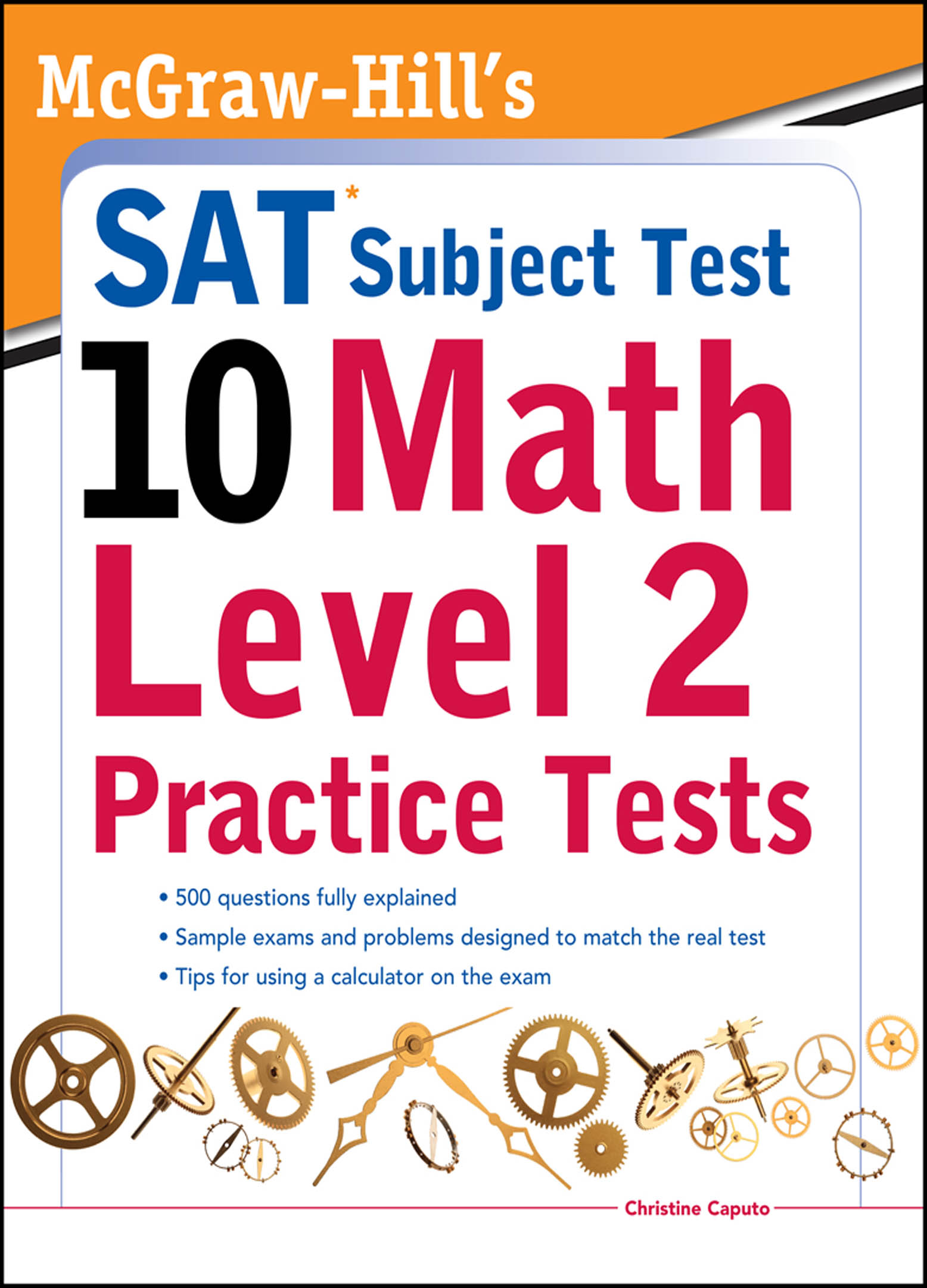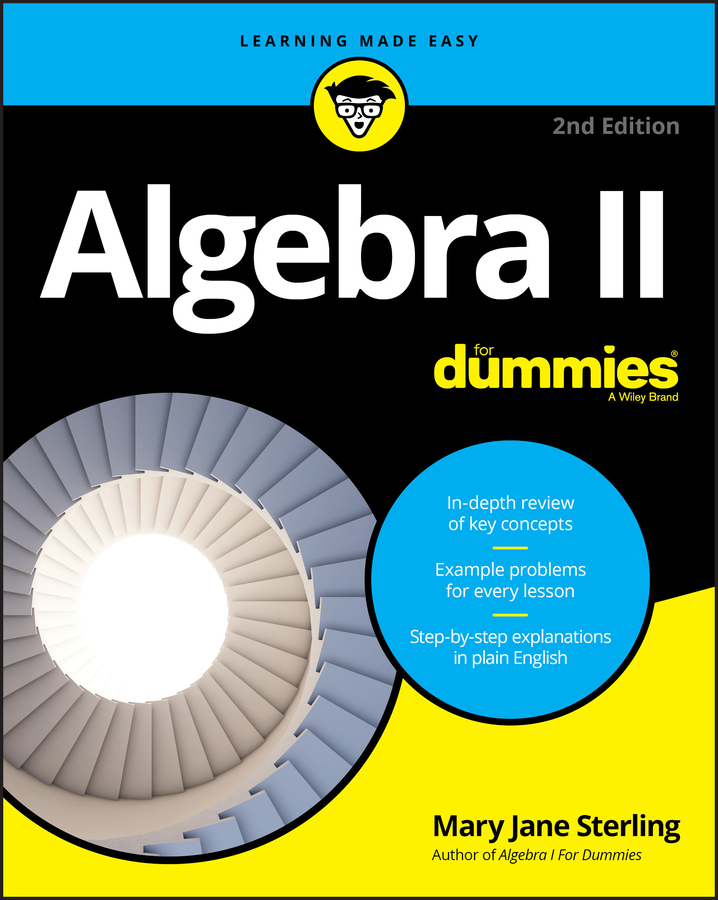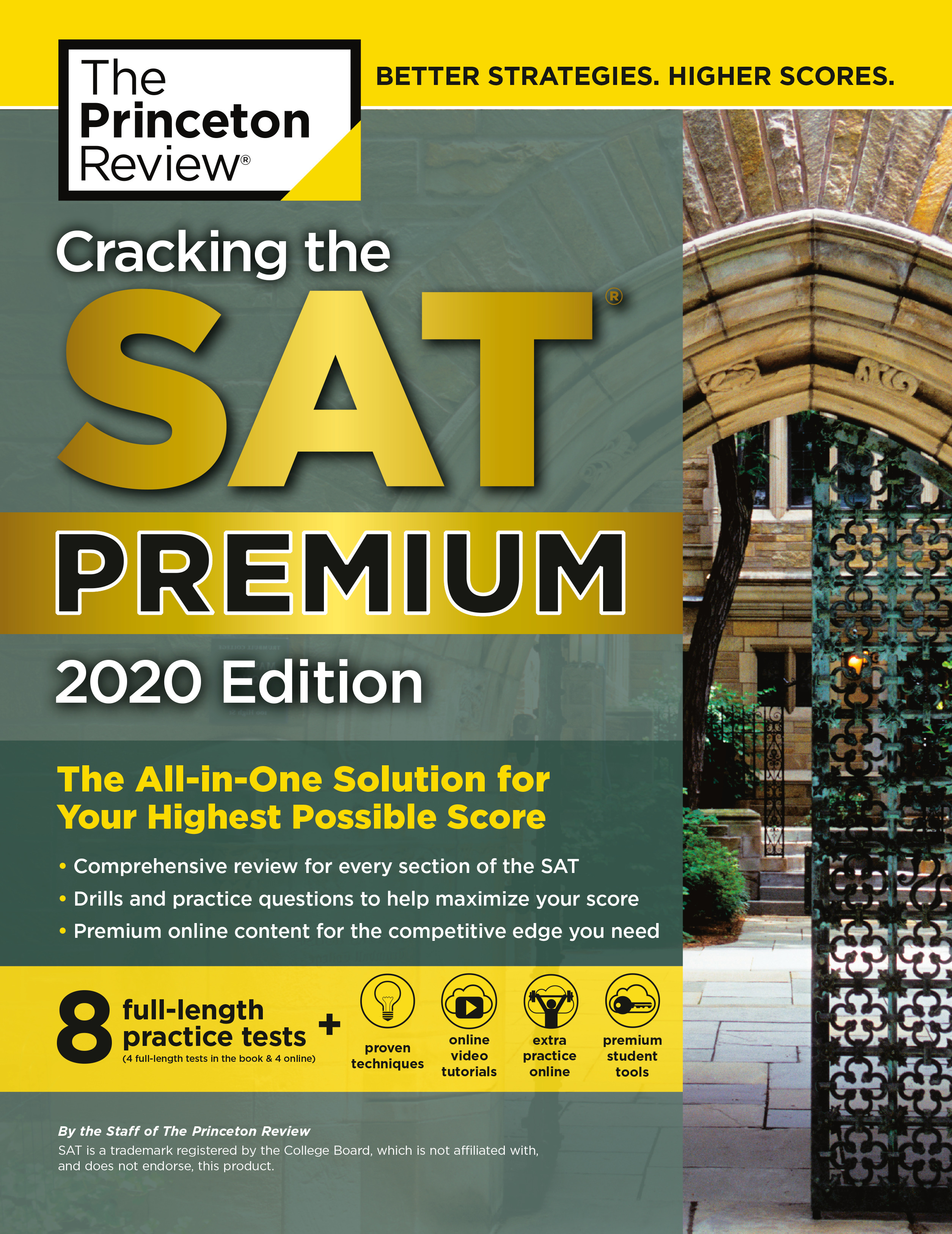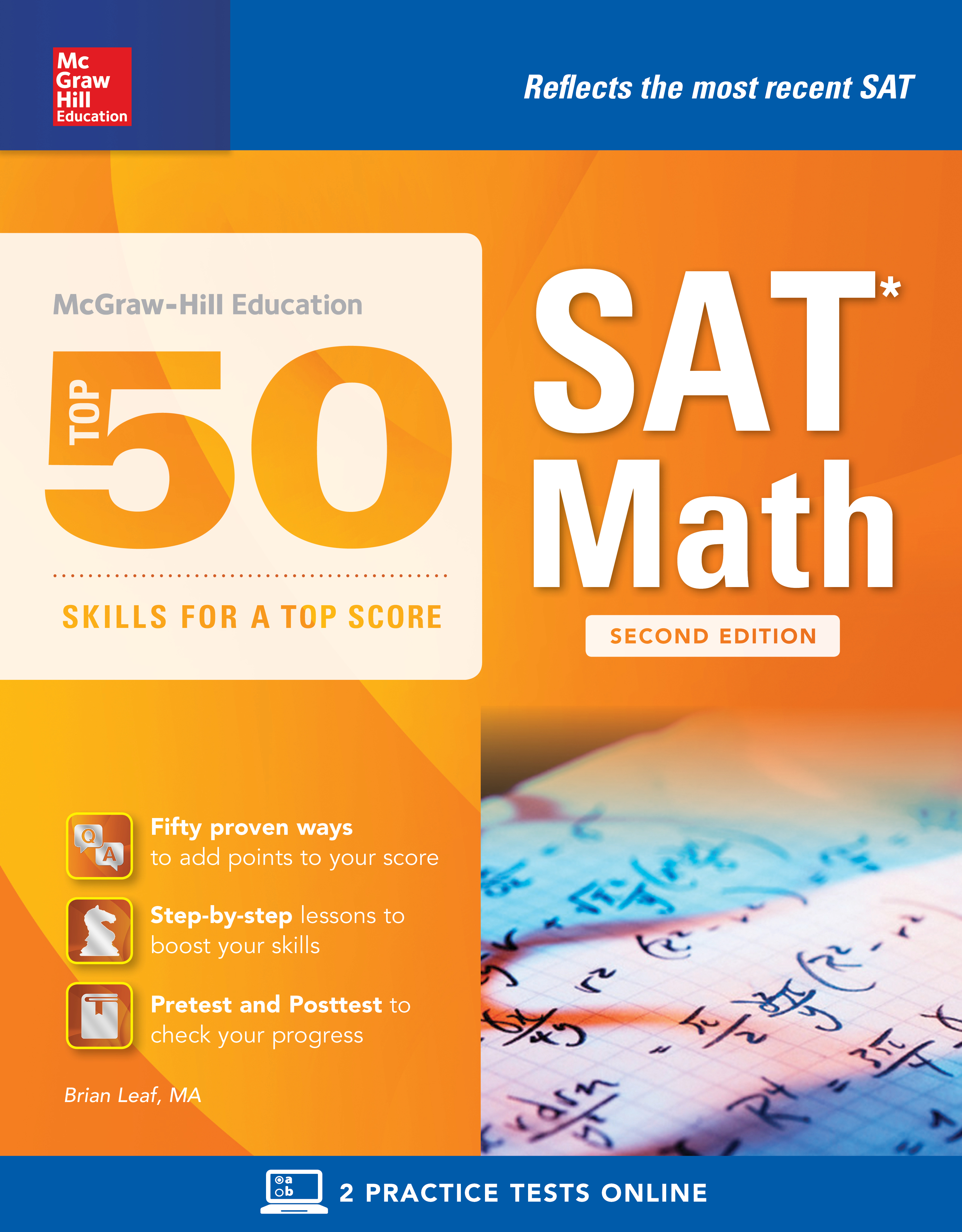Paperback Textbooks (New and Used)Sale of the Day!Overview of the SAT Math Content
 SAT Math 1Numbers and Operations  Ratio and Proportions, Counting Elementary Number TheoryComplex Numbers, Matrices, Sequence  Algebra and Functions Expressions, Equations, InequalitiesRepresentation and Modeling Properties of Functions (linear, polynomial, rational exponential) Geometry and Measurement Plane Euclidean  Coordinate (Lines, Parabolas, Circles, Symmetry Transformations) Three-dimensional (Solids, Surface Area and Volume) Trigonometry: (Right Triangles, Identities) Data Analysis, Stats, and Probability Mean, Median, Mode, Range, Interquartile Range Graphs and PlotsLeast Squares Regression, Probability  More on the SAT Math Level 1 Content SAT Math 2Numbers and Operations Operations, Ratio and Proportions, Complex Numbers Counting, Elementary Number Theory, Matrices Sequences, Series, Vectors Algebra and Functions  Expressions, Equations, inequalities, Representation and Modeling  Properties of Functions (Linear, Polynomial, Rational Exponential, Logarithmic, Trigonometric, Inverse Trigonometric, Periodic, Piecewise Recursive, Parametric) Geometry and Measurement Coordinate (Lines, Parabolas, Circles, Ellipses, Hyperbolas, SymmetryTransformations, Polar Coordinates)  Three-dimensional (Solids, Surface Area and Volume of Cylinders, Cones, Pyramids Spheres and Prisms along with Coordinates in Three Dimensions) Trigonometry: (Right Triangles, Identities, Radian Measure Law of Cosines, Law of Sines, Equations Double Angle Formulas) Data Analysis, Statistics, and Probability Mean, Median, Mode, Range, Interquartile Range, Standard Deviation, Graphs and Plots, Least Squares Regression (Linear, Quadratic, Exponential), Probability  More on the SAT Math Level 2 Content

 Decay:  The value of a certain machine has an annual decay factor of 0.75. If the                          machine is worth \$10,000 after 5 years, what was the original value of the                   machine?   The machine will decay exponentially as in radioactive elements.  Therefore, applying the Exponential Decay Formula:                                                     y = abx  where y = the final amount, a = the original value, b the decay factor,   and x the time in years.                           By substitution, 10,000 = (a)(0.75)5                                                        10,000 = (a)(0.2373)                                                                 a =  10000                                                                        0.2373                                                                    =  42,140.75                                                                        Answer: \$42,140.75 Radicals: Simplify √50 + √2 - 2√18 + √8.      50 + √2 - 2√18 + √8 = √(25 x 2) + √2 - 2√(9 x 2) + √(4 x 2)                                            = 5√2 + √2 - (2 x 3)√2 + 2√2                                           = 6√2 - 6√2 + 2√2                                           = 2√2                                  Answer: 2√2for Math Practice,  SAT  ACT

 More School Supplies and other useful items *  * *